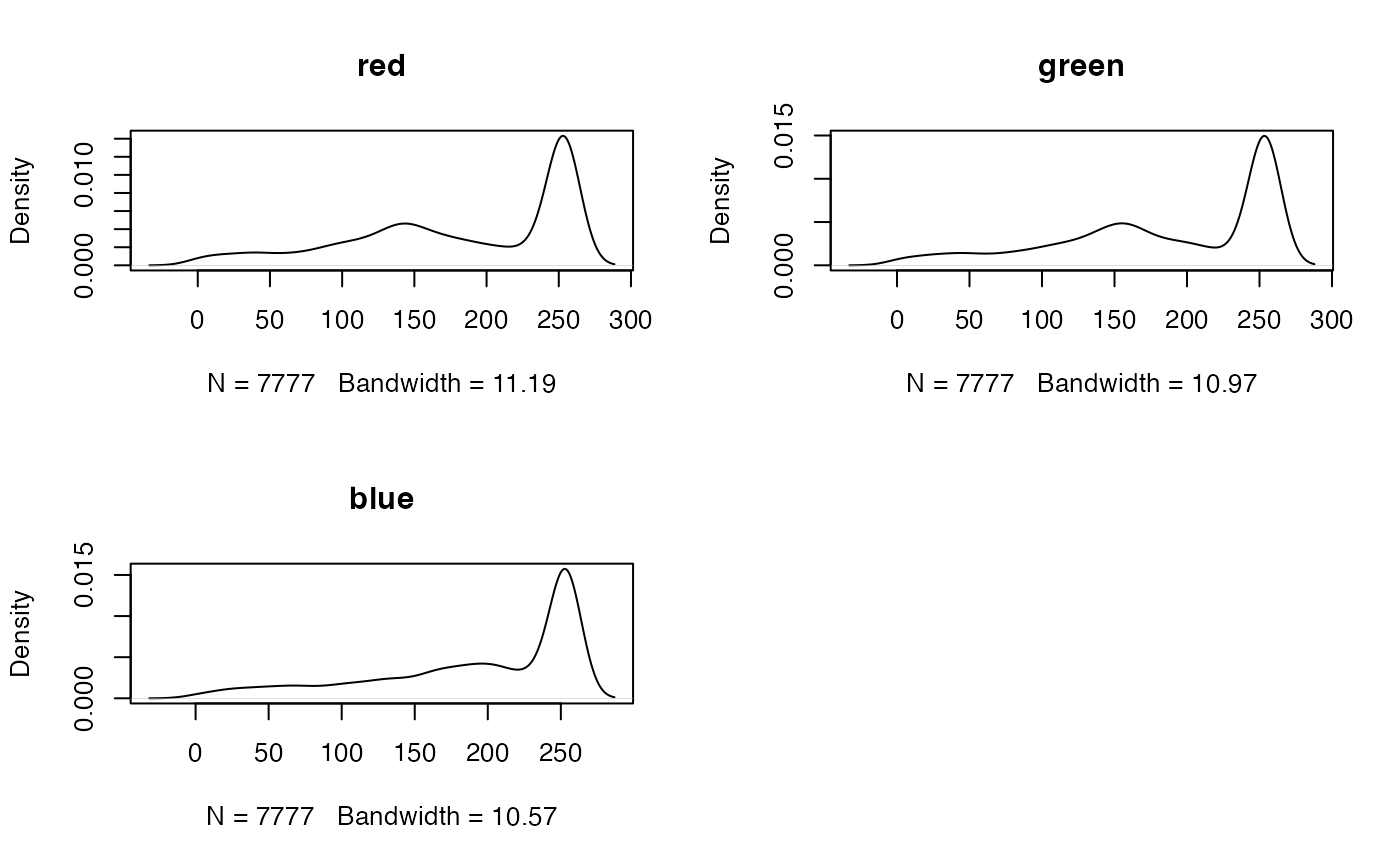Create density plots of values in a Raster object

# S4 method for Raster
density(x, layer, maxpixels=100000, plot=TRUE, main, ...)

## Arguments

x

Raster object

layer

numeric. Can be used to subset the layers to plot in a multilayer object (RasterBrick or RasterStack)

maxpixels

the maximum number of (randomly sampled) cells to be used for creating the plot

plot

if TRUE produce a plot, else return a density object

main

main title for each plot (can be missing)

...

Additional arguments passed to base plot

## Value

density plot (and a density object, returned invisibly if plot=TRUE)

## Examples

logo <- stack(system.file("external/rlogo.grd", package="raster"))
density(logo)